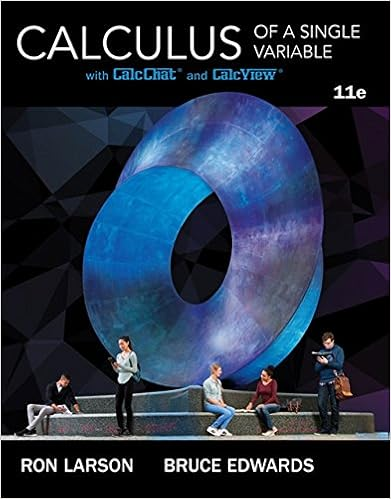# Michael_ShawMATH260_W6_Lab_Worksheet - MATH260Week 6 Lab...

• Lab Report
• 11
• 80% (5) 4 out of 5 people found this document helpful

This preview shows page 1 - 3 out of 11 pages.

##### We have textbook solutions for you!
The document you are viewing contains questions related to this textbook.The document you are viewing contains questions related to this textbook.
Chapter 5 / Exercise 54
Calculus of a Single Variable
Edwards/LarsonExpert Verified
MATH260—Week 6 LabName: Michael ShawAntiderivatives According to the first part of the fundamental theorem of calculus, the antiderivative reverses the derivative.If f(x) is a derivative, F(x) is the antiderivative.Directions:Look at the examples below and answer questions 1 and 2.Let f(x) be a derivative and F(x) be the anitderivative.a.)f(x) = 3x2b.)f(x) = 5x – 63
C) F(x)=xn+1/x+1.Set each term individually equal to their generic derivative equivalency d/dx=axnTake the Derivative of the generic termSet the coefficients equal to other like terms and solve for aUse a as the coefficient on the anti-derivative term along with the viable term and set all equal to F(x).2) Find the antiderivative of f(x) = 3x5+ x – 4. Show all work.
##### We have textbook solutions for you!
The document you are viewing contains questions related to this textbook.The document you are viewing contains questions related to this textbook.
Chapter 5 / Exercise 54
Calculus of a Single Variable
Edwards/LarsonExpert Verified
4) What do the derivatives of the following antiderivativeshave in common?
– 11
•••